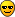# RooFit: chi^2 on plots and pullhistos

Would anyone have a code snippet that:

1. puts a chi^2 on a roofit plot

2. puts residuals (pullhistogram) underneath a the fit ?

james

Hi,

To calculate a chiSquare from a curve and a histogram in a RooPlot, simple call

Double_t chi2 = frame->chiSquare() ;

If multiple curves and or histograms are present you need to indicate which chisq you want to calculate by providing the names of the curve and histogram to the chiSquare() function. (You can set names of curves and histos by adding a Name(“blah”) argument to each plotOn call.

Concerning a residual or pull histogram, you can do the following. Given a RooPlot with one histogram and one curve named frame, you can make a second RooPlot with the residual or pull distribution as follows

``````RooPlot* frame2 = x.frame() ; // same way you made 'frame'
frame2->setMinimum(-5) ;
frame2->setMaximum(+5) ;
frame2->Draw() ;
``````

The pullHist() function will generate a histogram with pulls, the residHist() function will generate a histogram with residuals (i.e. not normalized w.r.t error)

Wouter

I am able to find my chi2 but I can’t put this on a plot. I try:

frame->Draw()
Double_t signalchi = frame->chiSquare();
TPaveLabel t1(0.7,0.6,0.9,0.68, Form("#chi^{2} = %f", singalchi));
t1->Draw();

This works on a general TCanvas. Is there something special I have to do to put TPaveLabels on a roofit plot?

james

```TPaveLabel t1(0.7,0.6,0.9,0.68, Form("#chi^{2} = %f", singalchi)); ```do

```TPaveLabel *t1 = new TPaveLabel(0.7,0.6,0.9,0.68, Form("#chi^{2} = %f", singalchi)); ```
otherwise your object will be deleted when your function goes out of scope.

Rene

Hi,

That will work (using the ‘new’ operator as suggested by Rene). You can also make the TPaveText part of the RooPlot by adding

This will make sure that

• Whenever you do frame->Draw() the pavetext is also (re)drawn
• When you save the RooPlot in a TFile, the TPaveText is persisted too.

Wouter

Hi all,
I tried to follow the recipies in this post but I failed.
Here is the relevant part of the code;

``````   /* ... */
hName.Form("Residual U for CS = 1, bin %i",i);
RooDataHist uData_CS1("uData_CS1",hName.Data(),u,ResU_Vs_PH_CS1[i]);
RooPlot* uFrame = u.frame();
uData_CS1.plotOn(uFrame);

RooFitResult* r_ml_wgt = uFcn.fitTo(uData_CS1,Extended());
uFcn.plotOn(uFrame);
uFcn.paramOn(uFrame,Format(("NEU"),AutoPrecision(1)),Layout(.6,.99,.99));
c1->SetLogy(log);

RooPlot* uFrame2 = u.frame();
Double_t signalchi = uFrame2->chiSquare();
outlog << "signal chi2 for bin " << i << " = " << signalchi << "\n";
t1[i] = new TPaveLabel(0.1,0.1,0.6,0.28, Form("#chi^{2} = %f", signalchi));
t1[i]->Draw();

uFrame->Draw();
saveNamepng.Form("plots/ResU_Vs_PH_CS1_Run%d.ps",runNumber);
c1->SaveAs(saveNamepng.Data());
``````

By the way, the value for signalchi is always -1.

I just want to print on frame the chi2 (or -ln(L)) or have some goodness-of-fit value to show on the frame.

Any help is welcome!

Regards,
Marco Bomben[/code]
batchAnalysis_rf4.c (4.48 KB)

You must draw the label in normalized device coordinates [0,1], ie

```TPaveLabel *t1 = new TPaveLabel(0.7,0.6,0.9,0.68, Form("#chi^{2} = %f", singalchi),"brNDC"); ```
Rene

Hi Rene.
thanks for quick reply. This fixed TPaveLabel issue.

I still get chi2 = -1.

Here’s the relevant part of code I’m using:

``````    RooFitResult* r_ml_wgt = uFcn.fitTo(uData_CS1,Extended());
uFcn.plotOn(uFrame);
uFcn.paramOn(uFrame,Format(("NEU"),AutoPrecision(1)),Layout(.6,.99,.99));
c1->SetLogy(log);

hName.Form("_resU_Vs_PH_%i_CS1_Det4",i);
Double_t signalchi = uFrame->chiSquare("uPdf",hName.Data(),6);
outlog << "signal chi2 for bin " << i << " = " << signalchi << "\n";
t1[i] = new TPaveLabel(0.1,0.1,0.3,0.28, Form("#chi^{2} = %f", signalchi),"brNDC");
t1[i]->Draw();

uFrame->Draw();
saveNamepng.Form("plots/ResU_Vs_PH_CS1_Run%d.ps",runNumber);
c1->SaveAs(saveNamepng.Data());``````

In attachment the whole code.

Many thanks again.
Marco
batchAnalysis_rf4.c (4.48 KB)

Hi all,
I was able to get proper chi2.
Sorry for the noise.

Regards,
Marco Bomben

Hello Rooters

I was trying to follow this thread for putting chi2 in the stats box in plot.
I have a script, which I compile outside root using g++. I want to put all the parameters on plot(After fiting),along with chi2.
To calculate chi2, and to put the value on plot, I do the following(*), it prints the chisquare, but after that
code got stuck, and the plot also doesn’t appear.

Could someone let me know if I am doing something wrong while using TPaveLabel, as if I remove this
TPaveaLabel part of the code, then things works fine, and I get the statistics box on the plot with the values
of other parameters after the fit.

Regards
Bhawna Gomber

(*)

RooPlot* frame = x.frame(Title(“M_{eg}”)) ;
dh.plotOn(frame,Name(“dh”)) ;
model.fitTo(dh,Extended(),Range(60,120)); model.plotOn(frame,Name(“model”));
model.plotOn(frame,Components(shape),LineStyle(kDashed)) ;

model.paramOn(frame,Layout(0.55),Format(“NEU”,AutoPrecision(1))) ;
Double_t chi2 = frame->chiSquare(“model”, “dh”,;
std::cout<<“Chi Square=:”<<chi2<<std::endl;

TPaveLabel *t1 = new TPaveLabel(0.7,0.6,0.9,0.68, Form("#chi^{2} = %f", chi2));
t1->Draw();
c->SaveAs(“try.png”) ;
inputfile->Close();

Hi,

Since this is RooFit related (and added to an old thread), I recommend you repost this issue in the Math and Stats forum.

Cheers,
Philippe.